# RSoft Application Gallery Note: Simulation of Twisted Nematic (TN) Liquid Crystal Cell

## Introduction

Twisted Nematic (TN) Liquid Crystal structures can be used to provide achromatic polarization rotation in thin layers, and play important roles in many optical applications such as display panels, modulators, and switches. The optical properties of a twisted TN cell between two polarizers have been theoretically analyzed and experimentally measured . This application note studies the well-known example of transmission through a 90 degree TN cell as a function of the cell thickness, as shown in Fig.1.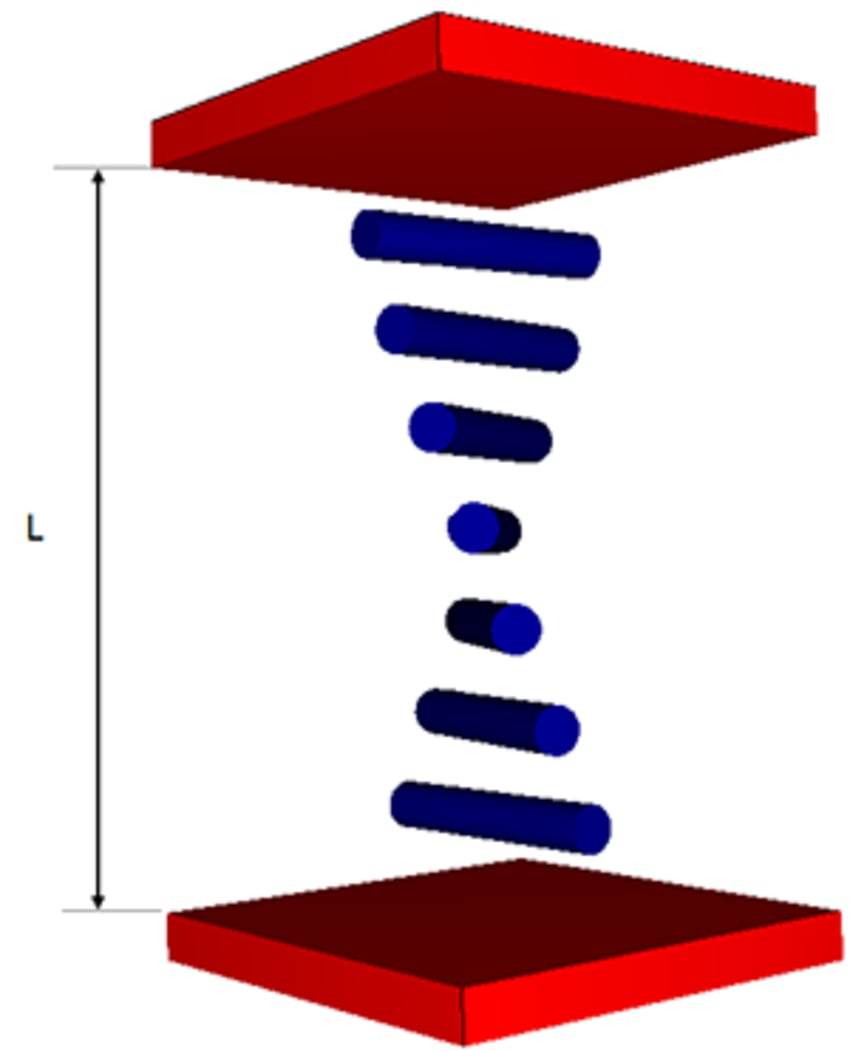Figure 1: Illustration of Twisted Nematic (TN) cell.

## Structure overview

To define this TN Liquid Crystal structure, we will utilize a base anisotropic material TN having the following refractive index tensor: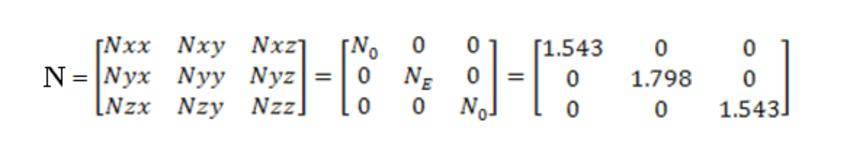The TN cell, as drawn in the RSoft CAD Environment™, is shown in Figure 2.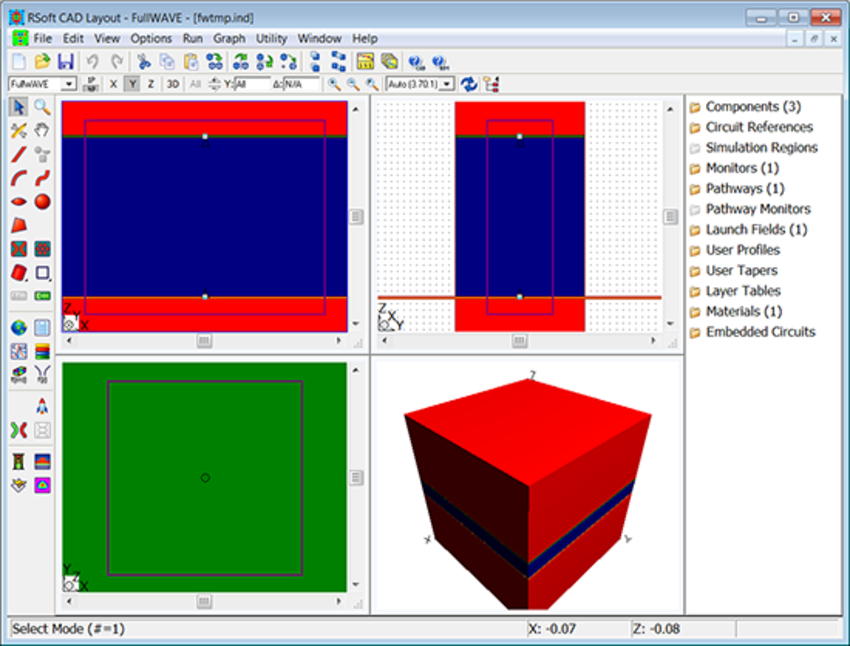Figure 2: The TN cell.

This structure is composed of three segments, all of which are defined using the same base TN anisotropic material. The crystal axis of this material is rotated around the Z axis to produce the TN structure, and is matched between the segments to create a uniform material. The crystal axis of each segment is rotated around the Z axis (Psi) as follows:

• Input Segment (bottom red)
This segment is defined via the TN material with a constant Crystal Axis orientation (Psi = 0 degrees).

• TN Segment (dark blue)
This segment is defined via the TN material with a linearly tapered Crystal Axis orientation (0 → Psi → 90). Later, we will study the transmission of the device as a function of the length of this segment.

• Output Segment (top red)
This segment is defined via the TN material with a constant Crystal Axis orientation (Psi = 90 degrees).

The excitation field is a TE planewave with a wavelength of 0.6328μm. Our aim is to measure the transmitted power in the TE field after it passes through the TN segment. We can realize this by using a monitor to measure the Ex field at the end of the middle twisted segment, and a metric in RSoft's MOST™ to calculate the power.

## Simulation Results

Using FullWAVE and MOST, we measure the transmittance of the device as a function of its length for the incident TE planewave field.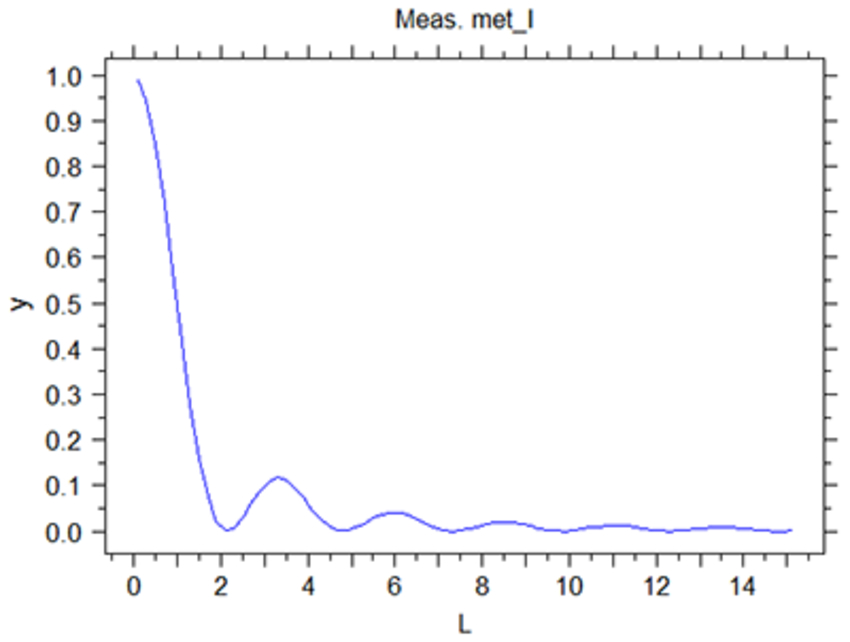Figure 3: Calculated Transmittance vs. TN cell length.

To obtain the typical plot of the Transmittance vs. the Mauguin parameter u = πLΔn/(λθ), we can scale the X axis of Figure 3 to obtain the plot shown in Fig 4. From these graphs, one can clearly see the 1st and 2nd Mauguin conditions, and also how well the simulation matches with the theoretical analysis.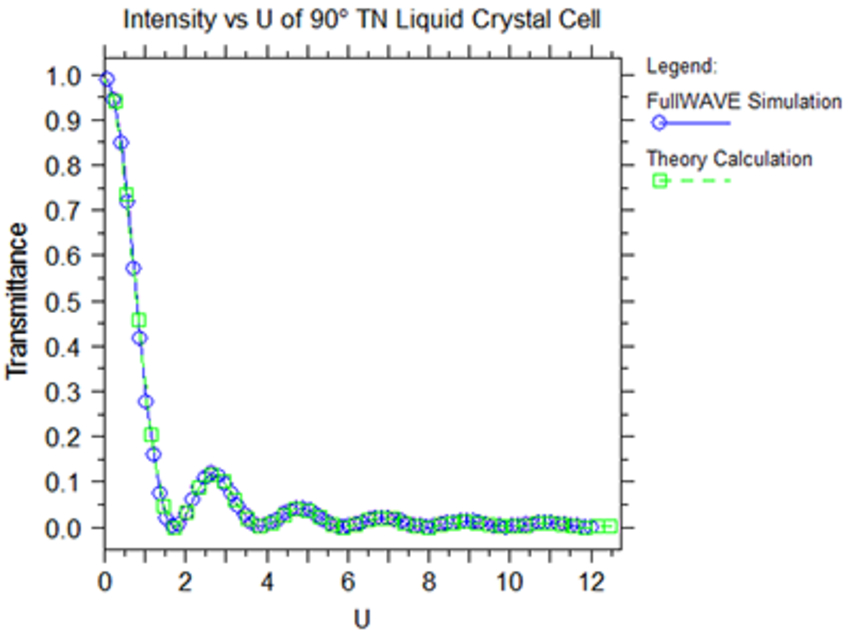Figure 4: Plot of the Transmittance vs. Mauguin parameter u (blue).
Also shown is the predicted Transmittance (green) calculated using Equation 1 in Ref. 1.

## References:

 Golovin et al, Achromatic Linear Polarization Switch for Visible and Near Infrared Radiation Based on Dual-Frequency Twisted Nematic Cell, Proc. of SPIE Vol.6135,61350E (2006)